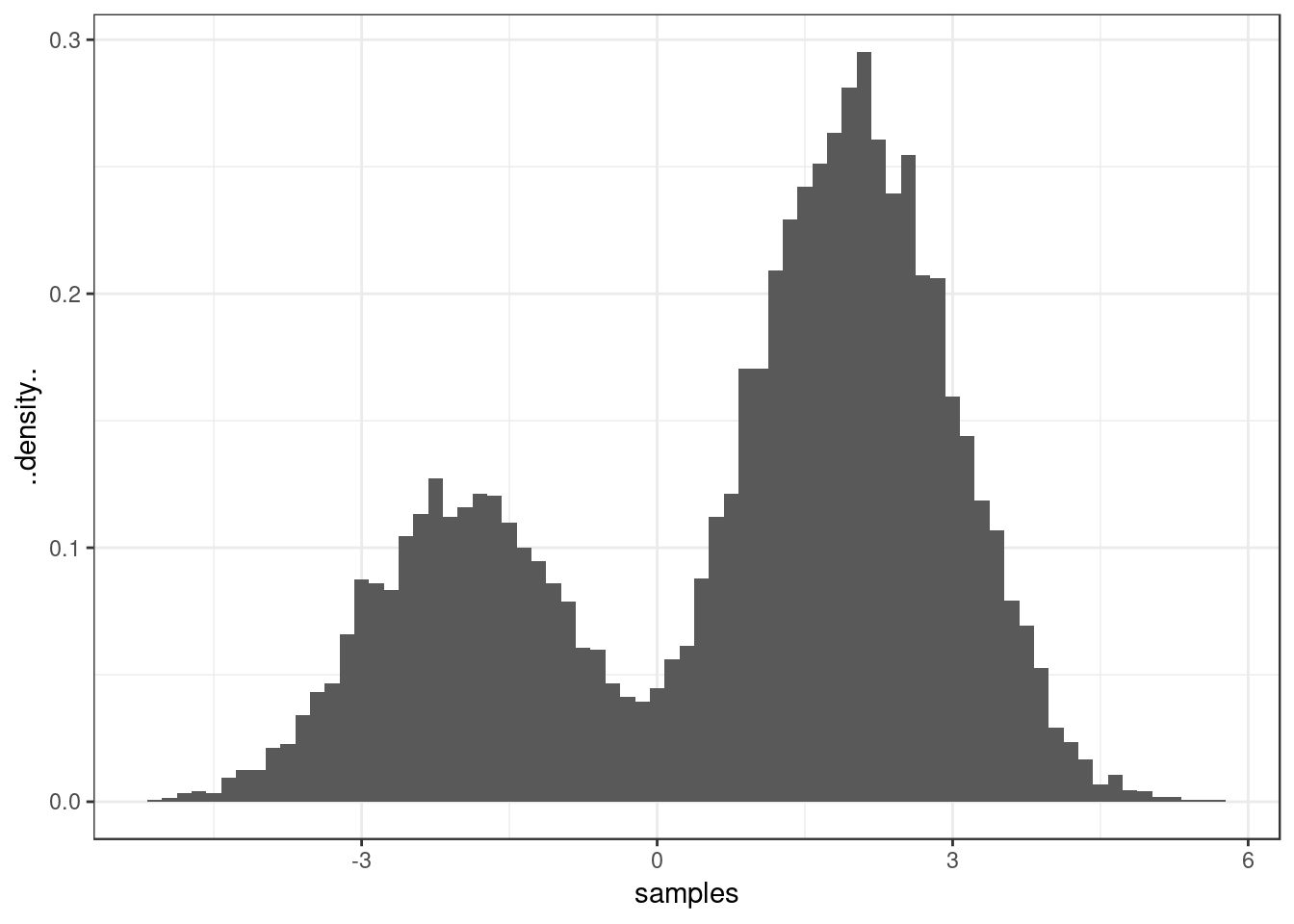### Mixture models

Let us introduce a mixture of two gaussian distributions with density $f(x) = \omega_1 \varphi(x; \mu_1, \sigma_1^2) + \omega_2 \varphi(x; \mu_2, \sigma_2^2)$ where $$\omega_1 = 1 - \omega_2 \in [0,1]$$, $$\mu_1,\mu_2 \in \mathbb{R}$$, $$\sigma_1^2, \sigma_2^2 \in \mathbb{R}^+$$ and $$\varphi$$ is the Gaussian density $\varphi(x; \mu, \sigma^2) = \frac{1}{\sqrt{2\pi\sigma^2}} \exp\left\{-\frac{1}{2\sigma^2}(x-\mu)^2\right\}$

How to draw a sample from the mixture distribution?

sample_mixture <- function(size, omega, mean, sd) {
# First sample indices which determine the Gaussian distribution used for
# each sample.
allocations <- sample(x = c(1, 2), size = size, replace = TRUE, prob = omega)

# Sample normal variates using the indices into the mean and variance
# vectors.  Here we are giving rnorm vectors of length n for the mean and
# standard deviation. In this case it generates each sample wit the mean and
# variance at the corresponding index.
rnorm(n = size, mean = mu[allocations], sd = sigma[allocations])
}

Let us set $$\omega_1 = 0.3 = 1 - \omega_2$$, $$\mu_1 = -2$$, $$\mu_2 = 2$$, $$\sigma_1^2 = \sigma_2^2 = 1$$ and run our sampler.

num_samples <- 10000
omega <- c(0.3, 0.7)
mu <- c(-2, 2)
sigma <- c(1, 1)

samples <- sample_mixture(num_samples, omega, mu, sigma)

# Plot a histogram of the samples
library(ggplot2)
theme_set(theme_bw())
qplot(x = samples, y = ..density.., geom = "histogram", binwidth = 0.15)